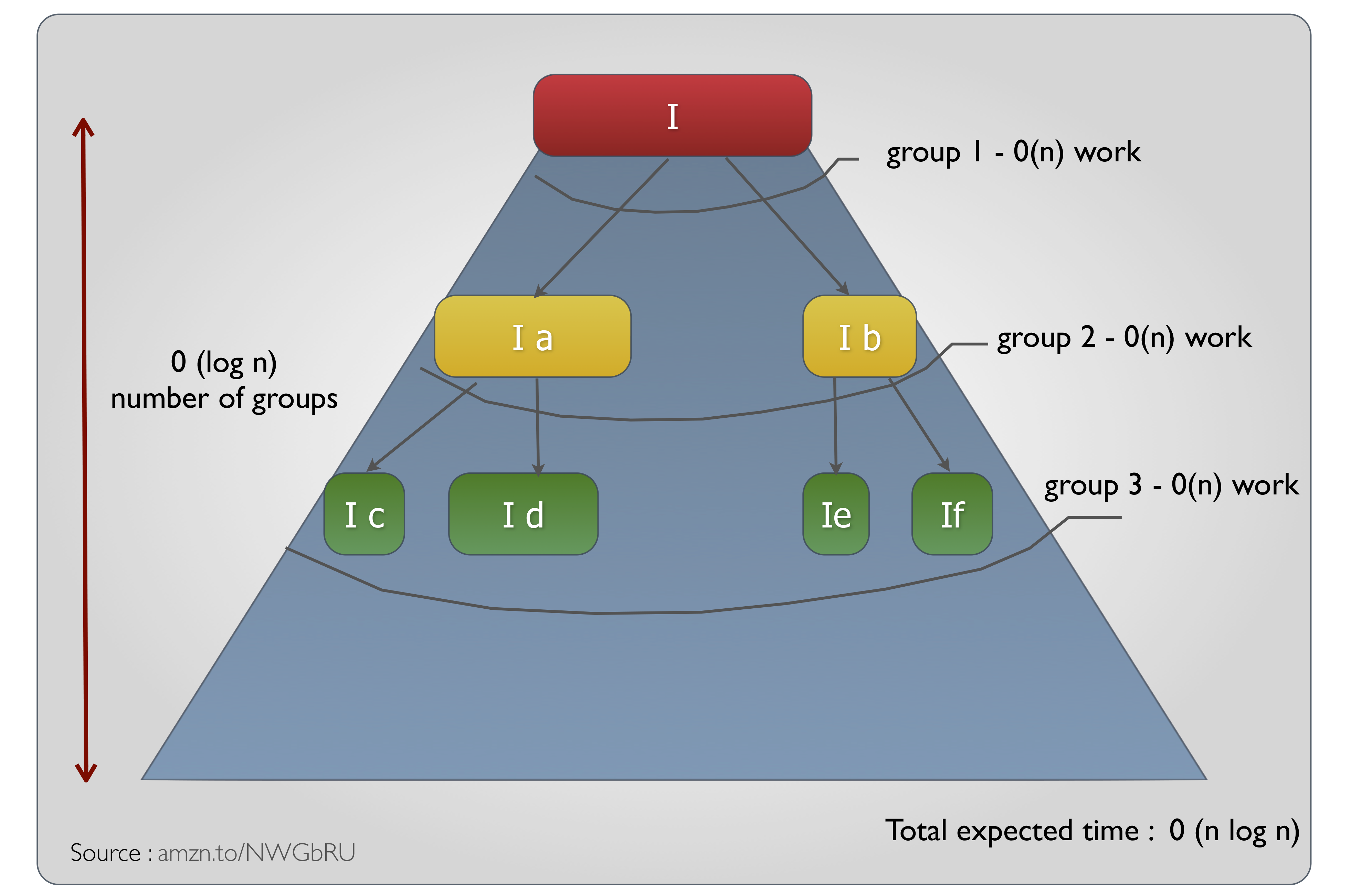Note : For 3-way partition and Dual Pivot Quicksort (with programs to trace the sort process), please refer to this recent post.

Quick sort is the fastest known non-hybrid comparision sort for arrays which has no knowledge of the data beforehand. To top it, it could be done in-place for arrays. For Linked Lists, Merge Sort might be a better option. Also since Quicksort improves its performance by using random numbers, it also becomes an example of a breed of algorithms named "Randomized Algorithms".

The naive version of the Algorithm goes like this :

``````1)	Start with list I of n items
2)	Choose pivot v from I
3)	Partition I into 2 unsorted lists I1 and I2 such that
I1 : all keys smaller than pivot
I2 : all keys larger than pivot
Items same as pivot goes to either list
4)	Sort I1 recursively, yielding sorted list S1
5)	Sort I2 recursively, yielding sorted list S2
6)	Concatenate S1,v,S2 yielding sorted list S
``````

Pseudocode in a Video

How Long does Quick sort take ?

Interestingly, Quicksort has a worst case running time of 0(n^2). However, with careful implementations, n^2 never happens and it almost always runs in 0(n log n). As compared to Heapsort, whose best and worst case is guaranteed to be n log n, Quicksort beats Heapsort in clock time for two reasons :

1. The constant factor surrounding the 0(nlogn) is lesser than Heapsort due to the very thin core logic. Heap sort's detailed comparison and exchange mechanism inside loops increases the constant factor.

2. Quicksort could leverage on the locality of reference (and therefore, memory access) advantages provided by the hardware and the operating system

How n log n?

If you choose the pivot right, then, on an average, you get a 1/4, 3/4 split which gives an average running time of 0(n log n)Source : Goodrich and Tamassia

Pivot Choosing strategy :

There are many methods to choose a pivot

``````1)	First Item
2)	Last item
3)	Median
4)	Median of k (generally of 3)
5)	Random item
``````

Choosing a random key as the pivot is the preferred method because of the guaranteed worst case possibility with the other selection methods with following kinds of sequences :

``````1)	Presorted list
when chosing first item as your pivot in case of natural order
last item as your pivot in case of reverse order
2)	Repeated elements
3)	Unimodal sequences* (when median is chosen as pivot)
``````

Bottom line : When you choose a pivot in such a way that one portion of your split (either I1 or I2) becomes empty AND if you are doing it all through the list, then you are essentially doing the split N times.

Quicksort in Java

``````package me.rerun;

import java.util.Arrays;

public class QuickSort {

public static void quickSort (Comparable comparableArray[], int lowIndex, int highIndex){

//at least one item must exist in the array
if (lowIndex>=highIndex){
return;
}

int pivotIndex=getMedianIndexAsPivotIndex(lowIndex, highIndex);
//1) Choose pivot from the sublist
Comparable pivot=comparableArray[pivotIndex];
System.out.println("Pivot : "+pivot);
//2) Swap the pivot to the last item in the array
swapItemsWithIndices(comparableArray, pivotIndex, highIndex);

/*
Get the border indices sandwiching the unsorted items alone (ignore pivot (now, in the highIndex))
set 'i' to point to the item before the first Index
set 'j' to point to the item before pivot

Notice that this way, the following invariant gets maintained
all through the sorting procedure

a. All items left of Index 'i' have a value <=pivot
b. All items right of Index 'j' have a value >=pivot
*/

int i=lowIndex-1;
int j=highIndex;

do{ //Notice the <j (pivot item is ignored). We stop when both the counters cross

//compareTo will return 0 when it reaches the pivot - will exit loop
do {i++;} while (comparableArray[i].compareTo(pivot)<0);
//we dont have the protection as the previous loop.
//So, add extra condition to prevent 'j' from overflowing outside the current sub array
do {j--;} while (comparableArray[j].compareTo(pivot)>0 &&(j>lowIndex));

if (i<j){
swapItemsWithIndices(comparableArray, i, j);
}
System.out.println("I :"+i + " J :"+j);
} while (i<j);

swapItemsWithIndices(comparableArray, highIndex, i);//bring pivot to i's position
System.out.println("Comparable array : "+Arrays.asList(comparableArray));

//the big subarray is partially sorted (agrees to invariant). Let's recurse and bring in more hands

quickSort(comparableArray, lowIndex, i-1); //sort subarray between low index and one before the pivot
quickSort(comparableArray, i+1, highIndex); //sort subarray between low index and one before the pivot
}

//... since swapping with array is the easiest way to swap two objects
private static void swapItemsWithIndices(Comparable[] comparableArray, int firstItem, int secondItem) {
System.out.println("Swapping "+comparableArray[firstItem] +"  and  "+comparableArray[secondItem]);
final Comparable tempItem=comparableArray[firstItem];
comparableArray[firstItem]=comparableArray[secondItem];
comparableArray[secondItem]=tempItem;
System.out.println("After swap array : "+Arrays.asList(comparableArray));
}

//Variation 1 - chose median as pivot
private static int getMedianIndexAsPivotIndex(int lowIndex, int highIndex) {
return lowIndex+((highIndex-lowIndex)/2);
}

public static void main(String[] args) {

//Integer[] unsortedArray=new Integer[]{1,32,121,1424,2,1214,121214,3535,754,343};
//Integer[] unsortedArray=new Integer[]{4,4,8,0,8,9,7,3,7,6};
Integer[] unsortedArray=new Integer[]{5,5,5,5,5,5,5,5,5,5};

long startTime = System.nanoTime();
System.out.println("Original array : "+Arrays.asList(unsortedArray));
quickSort(unsortedArray, 0, unsortedArray.length-1);

System.out.println("Sorted array : "+Arrays.asList(unsortedArray));
System.out.println(System.nanoTime()-startTime);
}

}
``````

References